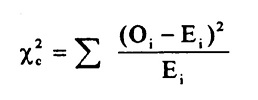# Chi – Square Test

### Chi – Square Test

There are two types of chi-square tests. Both use the chi-square statistic and distribution for different purposes:

1. Achi-square goodness of fit test determines if a sample data matches a population. For more details on this type, see: Goodness of Fit Test.
2. A chi-square test for independence compares two variables in a contingency table to see if they are related. In a more general sense, it tests to see whether distributions of categorical variables differ from each another.
• A very small chi square test statisticmeans that your observed data fits your expected data extremely well. In other words, there is a relationship.
• A very large chi square test statistic means that the data does not fit very well. In other words, there isn’t a relationship.The subscript “c” are the degrees of freedom. “O” is your observed value and E is your expected value. It’s very rare that you’ll want to actually use this formula to find a critical chi-square value by hand. The summation symbol means that you’ll have to perform a calculation for every single data item in your data set. As you can probably imagine, the calculations can get very, very, lengthy and tedious. Instead, you’ll probably want to use technology:

• Chi Square Test in SPSS.
• Chi Square P-Value in Excel.

A chi-square statistic is one way to show a relationship between two categorical variables. In statistics, there are two types of variables: numerical (countable) variables and non-numerical (categorical) variables. The chi-squared statistic is a single number that tells you how much difference exists between your observed counts and the counts you would expect if there were no relationship at all in the population.

There are a few variations on the chi-square statistic. Which one you use depends upon how you collected the data and which hypothesis is being tested. However, all of the variations use the same idea, which is that you are comparing your expected values with the values you actually collect. One of the most common forms can be used for contingency tables:Where O is the observed value, E is the expected value and “i” is the “ith” position in the contingency table.

A low value for chi-square means there is a high correlation between your two sets of data. In theory, if your observed and expected values were equal (“no difference”) then chi-square would be zero — an event that is unlikely to happen in real life. Deciding whether a chi-square test statistic is large enough to indicate a statistically significant difference isn’t as easy it seems. It would be nice if we could say a chi-square test statistic >10 means a difference, but unfortunately that isn’t the case.

You could take your calculated chi-square value and compare it to a critical value from a chi-square table. If the chi-square value is more than the critical value, then there is a significant difference.

You could also use a p-value. First state the null hypothesis and the alternate hypothesis. Then generate a chi-square curve for your results along with a p-value (See: Calculate a chi-square p-value Excel). Small p-values (under 5%) usually indicate that a difference is significant (or “small enough”).

Tip: The Chi-square statistic can only be used on numbers. They can’t be used for percentages, proportions, means or similar statistical value. For example, if you have 10 percent of 200 people, you would need to convert that to a number (20) before you can run a test statistic.

## 4 thoughts on “Chi – Square Test”

error: Content is protected !!